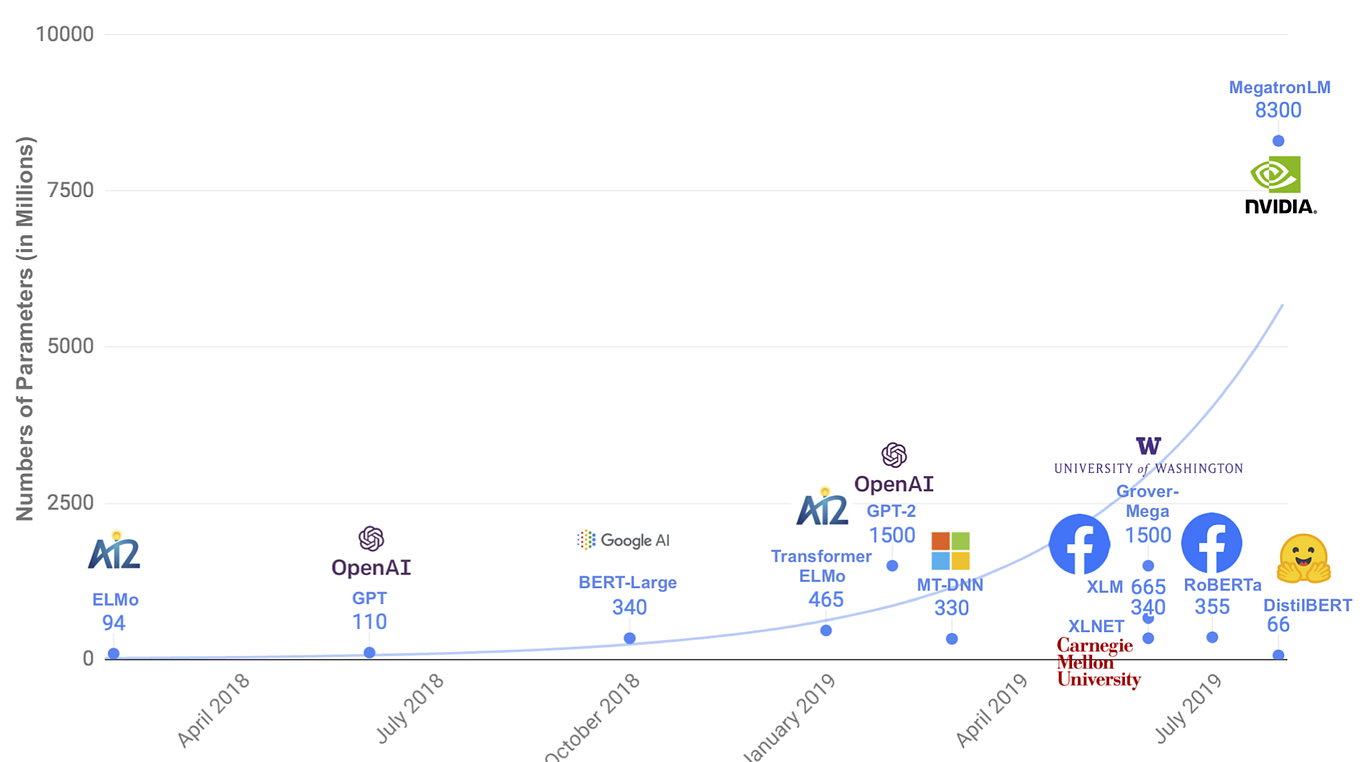### dair.ai

Democratizing Artificial Intelligence Research, Education, and Technologies

# DistilBERT — Half the price, same performance## Introduction

Recent publications within NLP present models with parameter counts that one after another continue to increase, now reaching parameter counts as high as in the tenths of billions (Google T5 with 11B parameters) While more parameters might be the key to optimal performance does it prevent model training and serving where the computational budget might be limited. The paper summarised in this article, “DistilBERT, a distilled version of BERT: smaller, faster, cheaper and lighter” address’ this issue through a method called Knowledge Distillation.

## Knowledge distillation

Knowledge Distillation, introduced by Bucila et al. (2006) and further improved by Hinton et al. (2015) (see appendix for comments on the contribution of these papers) uses a larger model as a teacher for a smaller one which tries to replicate its outputs and sub-layer activation for a given set of inputs. Previous work has shown that it is beneficial to consider more than just the true targets (the one-hot vectors) when training a student. Using the output distribution from the teacher over all possible targets can provide valuable information for creating a student with generalisability. For example, in predicting the hidden word in the sequence “I think this is the beginning of a beautiful [mask]”, the teacher might assign high probabilities to words like “day” and “life”. Relatively speaking might there also be high probabilities assigned to other words as “world”, “story” or “future”. This nuance is important for the student model to learn in order for it to generalise, rather than only learning the correct target. This paper capture this information through loss function used to train the student, comprised of a linear combination of the following three factors: Distillation loss. The output probabilities of the teacher (t) are combined with the ones from the student (s) as shown in the equation below. To minimise this loss does the student network therefore want to have higher certainty (probability) for the same outputs the teacher provided large probabilities. The teacher probabilities are calculated through temperature softmax, a modification to softmax introduced by Hinton et al. as a way to get more out of the granularities from the teacher model output distribution. It basically reduces the size of large probabilities while increasing that of the smaller ones, creating a smoother output distribution.Distillation lossTemperature softmax. z is the output logit from the teacher model while T is the temperature. A larger T creates a smoother output distribution.

Cosine embedding loss. A distance measure between hidden representations for student and teacher. This essentially enables the student to mimic the teacher in more layers than just the output layer, intuitively enabling creation of a better model

Masked Language Modelling loss. The same loss as used when training BERT to predict the correct token masked from the sequence.

## Architecture

The student network architecture is a Transformer encoder model with half the number of layers compared to BERT-base, while keeping the hidden representation dimension the same. This results in a total parameter count of 66M compared to the teacher model’s 110M. The reason for this choice is that reducing model size through the number of layers achieve the highest reduction in computation complexity. Reducing the hidden state representation (the size of the vectors) would due to linear algebra optimisations used for each vector operation have achieved the same reduction in model size but not in computational complexity. Further, simply changing the number of layers enables the student network to be initialised with parameters from every other layer in the teacher model.

## Training

This student network was trained on the same dataset as the original BERT model following best practices for transformer encoders; large batches (up to 4k samples) only tasked with predicting masked tokens and not the next sentence prediction task. Compared to RoBERTa could this distilled BERT model be trained with only 1.5% of the aforementioned models computational cost.

## Results

DistilBERT is able to achieve 97% of BERT-base’s score on the GLUE benchmark and 99.3% on the IMDb classification task. This while reducing model size and computational time with around 40%. An ablation study revival the importance of the different loss objectives, showing that both cosine distance between student and teacher hidden representations and distillation loss contribute the most to the overall student performance, increasing the GLUE score by about 4 points. Weight initialisation from the pre-trained model also prove to provide similar gains in performance, increasing the GLUE score by 4.83 when comparing to a distilled model trained without the initialisation.

## Conclusion

Knowledge distillation enables larger models, or ensembles of models, to be condensed into a single, smaller student network. This enables usage of state of the art architectures to be deployed where the computational environment is limited. With recent developments within the field of NLP, e.g. Google’s T5, do I believe that the area of knowledge distillation will continue to evolve over the years to come.

## Appendix

Bucila et al. distills an ensemble of models into a single student model. The authors also bootstraps the training data through processing unlabelled examples with the ensemble, enabling the student to more easily mimic its teachers. Hinton et al. introduces temperature softmax as part of the loss function which create softer targets for the student to mimic. These high entropy (close to an even distribution) provide much more information than the true targets would, enabling the student to more easily learn to generalise.

Original. Reposted with permission.

About the author: Viktor Karlsson is a Machine Learning Engineer with a growing interest of NLP. Trying to stay on-top of recent developments within the ML field in general, and NLP in particular, with the goal of sharing knowledge and understanding to a wider audience.